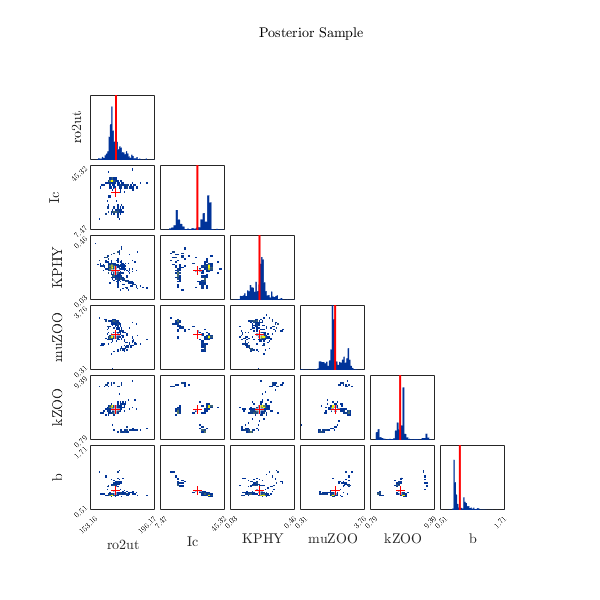# How to do Bayesian Inversion on a Kriging Metamodel

Hello UQWorld,

Some advice on my following problem would be much appreciated thanks.

I have an expensive ocean model which returns a single value indicating the difference between the modelled output ocean and the real/observed/true ocean. This model uses 6 parameter values in it’s partial differential equations, and I want to find the 6 parameters which results in a model output of ~zero (no difference between the modelled ocean and the real ocean).

I’ve replaced the expensive model with a Kriging meta model using uq lab, and did a Bayesian Inversion study using the attached script “Bayesian_Study_on_my_Kriging_Model.m”, which uses the attached function “my_ForwardBayesFunction.m” and zipped Kriging model “Kriging_MetaModel.mat”. The result looks ok (see attached figure “Posterior_Sample.png”).

What I would like is some advice on if I’ve done Steps 4 and 5 correctly in “Bayesian_Study_on_my_Kriging_Model.m”, as these are the sections I was most unsure on. The script should be well commented, but please let me know if I’m not clearThank you very much in advance,
Sophy

Kriging_MetaModel.mat.zip (1.5 MB) my_ForwardBayesFunction.m (248 Bytes) Bayesian_Study_on_my_Kriging_Model.m (2.4 KB)1 Like

Hi Sophy - welcome to UQWorld

1. Your Kriging metamodel looks fine and with a global validation error of \varepsilon_{\mathrm{val}}=1.8\% is probably sufficiently accurate to replace your original model, however, to ensure that the metamodel is sufficiently accurate near the point estimator \mathbf{x}_{\mathrm{MAP}} or the posterior mean, I would recommend that you rerun your original model at this point to ensure that it is also sufficiently accurate there;
2. The way you define your forward model greatly slows down the MCMC algorithm. You don’t need to reload Kriging_MetaModel.mat as part of the model definition. Instead, I suggest you load Kriging_MetaModel.mat once and pass it directly to the BayesOpts.Model field;

These are mostly efficiency issues, there are also two bigger issues with your code:

### Data

Typically, you would assign the data (i.e., your measurements) directly to the BayesOpts.Data.y field and not mix them with your model definition by letting the model output the difference between the two, but with as little work as possible in your case you would set the data to \mathcal{Y}=0. However, this implicitly assumes, that you only have a single measurement - is this true? Also, setting up your problem this way precludes you from using the default discrepancy options (see below).

### Discrepancy options

The discrepancy options specify the properties of the discrepancy between the forward model \mathcal{M} and the data \mathcal{Y} as

y = \mathcal{M}(\mathbf{X}) + \varepsilon

where \varepsilon\sim\mathcal{N}(0,\sigma^2). As you don’t know the proper value for \sigma^2, the Bayesian framework lets you infer the proper value together with the parameters of your forward model.

Note that we deemed your Kriging surrogate model sufficiently accurate and therefore neglect the difference between the actual forward model and the Kriging metamodel!

The simplest way to infer the discrepancy parameters would be not to define a DiscrepancyOptsKnown field and instead use the default options. I suggest you have a quick look at sections 1.2.3 and 2.2.6.2 of the module user manual for details.

In your case of \mathcal{Y}=0, however this will cause an error, because the default prior for \sigma^2 will be \mathcal{U}(0,\mu_{\mathcal{Y}}^2=0). I suggest you define the discrepancy options yourself and use a high enough upper bound for the uniform prior.

Anyways, I attach an updated version of your file Bayesian_Study_on_Kriging_Model, just let it run and tell me if you are happy with the results.

P.S.: We just released UQLab version 1.3 - be sure to check it out!

1 Like

Hi Paul,
Thank you so much for you reply - I didn’t expect one so soon! I’m off for a few days now but will try it when I get back then reply properly then.
Sophy

Hi Paul

Thanks again